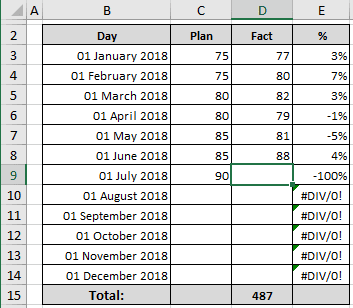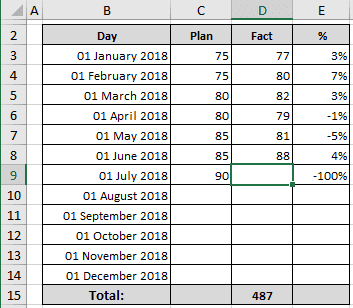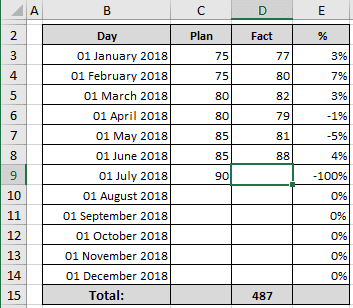# Hide formula errors in Excel

Excel
If you use formulas in Excel, you often can see messages such as #VALUE! or #DIV/0!. Most of the time, this means you need to check the source of the error and fix it, but sometimes a formula error simply means that the data used by the formula is not yet available.

For example, if you have a spreadsheet where you track monthly sales as a percentage:The formulas in column E do the calculations of percentages. For example, cell E3 contains the formula:

= (D3 - C3) / C3.

The formula in E4 was simply copied down the column to handle the other data.

The formula is correct for all cells, but in E10:E14, it is displayed the #DIV/0! error message, because of empty cells in C10:C14.

You can avoid displaying formula errors by changing the formula to use an IF and an ISERROR function:

= IF (ISERROR (<formula>), "", <formula>)

To display a blank if this formula returns an error. In this example:

= IF (ISERROR ((D3 - C3) / C3), "", (D3 - C3) / C3):If you prefer, you can replace the empty string ("") with zero or other text of your choice, just make sure the text is enclosed in quote marks. For example:See also this tip in French: Masquer les erreurs de formule dans Excel.